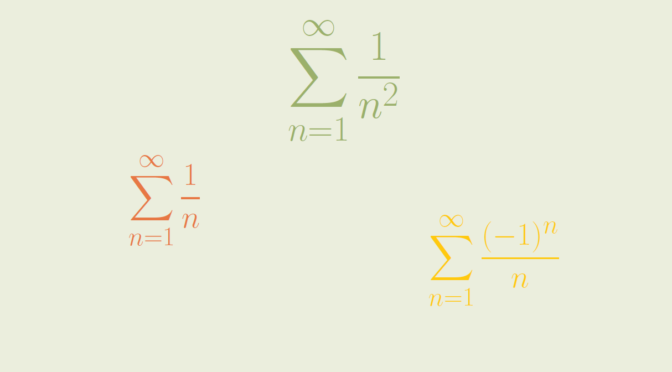# Ratio test

The ratio test is a test for the convergence of a series $\sum_{n=1}^\infty a_n$ where each term is a real or complex number and is nonzero when $$n$$ is large. The test is sometimes known as d’Alembert’s ratio test.

Suppose that $\lim\limits_{n \to \infty} \left\vert \frac{a_{n+1}}{a_n} \right\vert = l$ The ratio test states that:

• if $$l < 1$$ then the series converges absolutely;
• if $$l > 1$$ then the series diverges.

What if $$l = 1$$? One cannot conclude in that case.

### Cases where $$l=1$$ and the series diverges

Consider the harmonic series $$\displaystyle \sum_{n=1}^\infty \frac{1}{n}$$. We have $$\lim\limits_{n \to \infty} \frac{n+1}{n} = 1$$. It is well know that the harmonic series diverges. Recall that one proof uses the Cauchy’s convergence test based for $$k \ge 1$$ on the inequalities: $\sum_{n=2^k+1}^{2^{k+1}} \frac{1}{n} \ge \sum_{n=2^k+1}^{2^{k+1}} \frac{1}{2^{k+1}} = \frac{2^{k+1}-2^k}{2^{k+1}} \ge \frac{1}{2}$

An even simpler case is the series $$\displaystyle \sum_{n=1}^\infty 1$$.

### Cases where $$l=1$$ and the series converges

We also have $$\lim\limits_{n \to \infty} \left\vert \frac{a_{n+1}}{a_n} \right\vert = 1$$ for the infinite series $$\displaystyle \sum_{n=1}^\infty \frac{1}{n^2}$$. The series is however convergent as for $$n \ge 1$$ we have:$0 \le \frac{1}{(n+1)^2} \le \frac{1}{n(n+1)} = \frac{1}{n} – \frac{1}{n+1}$ and the series $$\displaystyle \sum_{n=1}^\infty \left(\frac{1}{n} – \frac{1}{n+1} \right)$$ obviously converges.

Another example is the alternating series $$\displaystyle \sum_{n=1}^\infty \frac{(-1)^n}{n}$$.